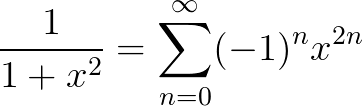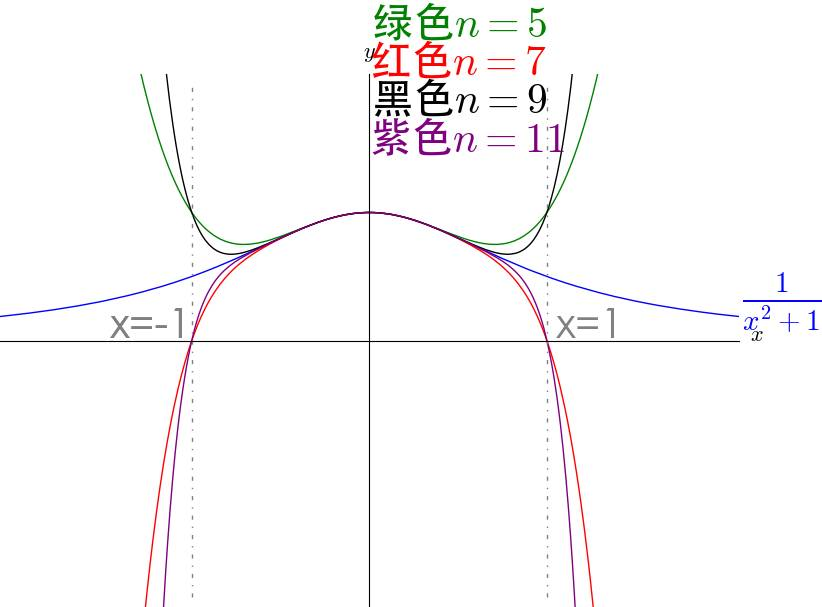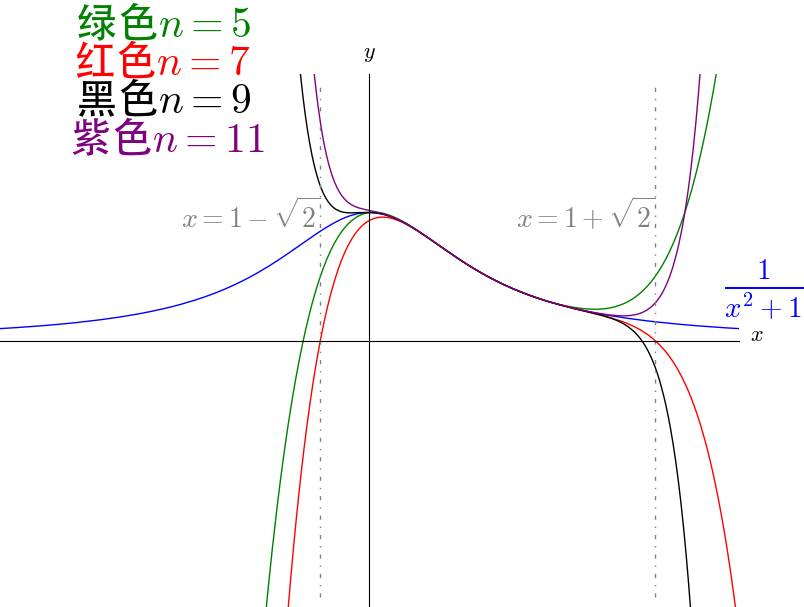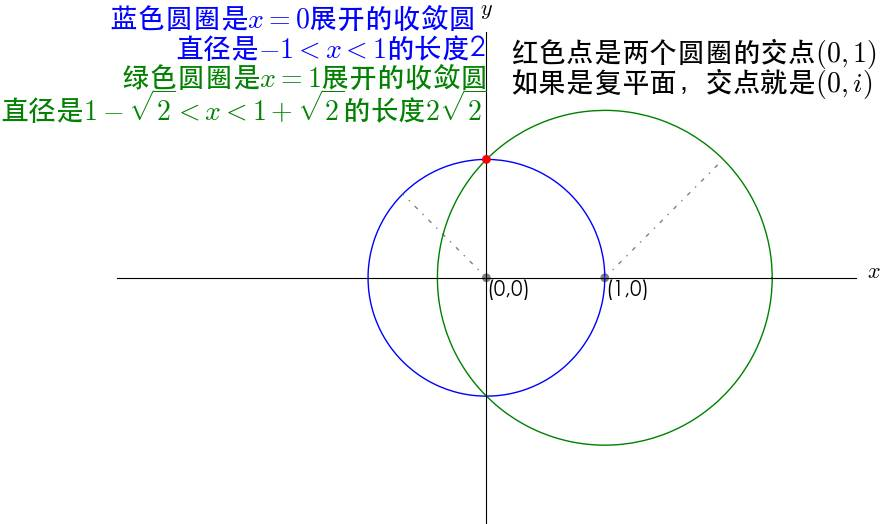# ZYMIN

0%

## 1 数系的扩展

### 1.1 整数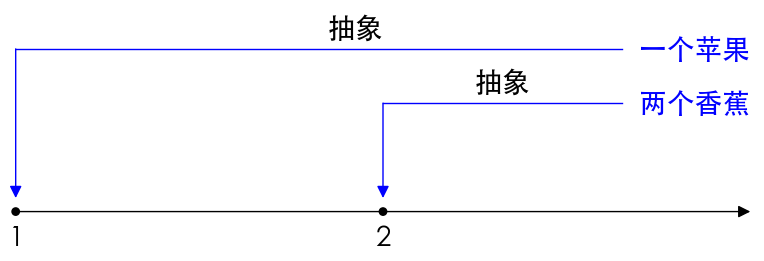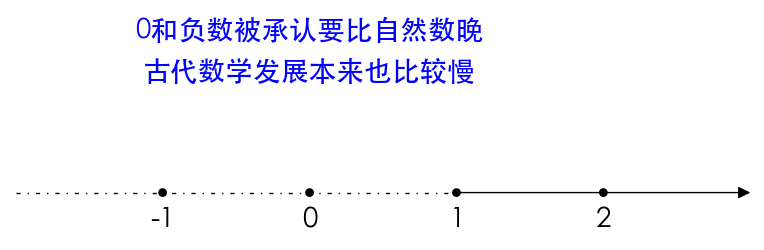### 1.2 有理数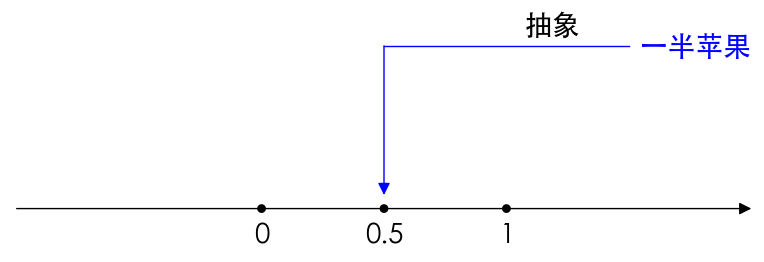### 1.3 无理数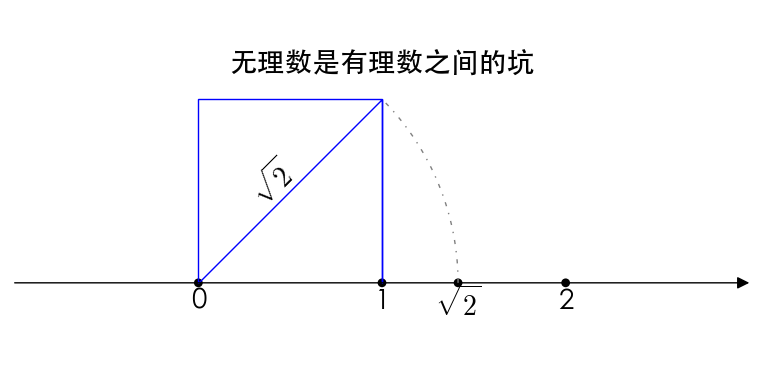$\sqrt2$是第一个发现的无理数，因此还引发了第一次数学危机。我们回头来看看 $\sqrt 2$ ，不通过证明我们还真没有办法说明它不是有理数，实际上大多数时候，无理数都需要证明，比如 e，$\pi$ 这样有名的无理数，在证明之前我们并不知道它是有理数还是无理数，而且证明难度还不小。## 2 虚数是否真是存在？

### 2.2 虚数似乎没有充分存在的理由

• 从自然数扩张到整数：增加的负数可以对应“欠债、减少”
• 从整数扩张到有理数：增加的分数可以对应“分割、部分”
• 从有理数扩张到实数：增加的无理数可以对应“单位正方形的对角线的长度（$\sqrt2$）
• 从实数扩张到复数：增加的虚数对应什么？

$$x= \frac {-b\pm\sqrt {b^2-4ac}}{2a}$$
，其判别式$\Delta=b^2-4ac$ 。

• $\Delta>0$有两个不等的实数根
• $\Delta=0$有两个相等的实数根
• $\Delta<0$有两个不同的复数根，

### 2.3 虚数是解一元三次方程的必须工具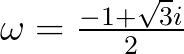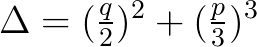，注意观察解的形式， $\Delta$ 是被包含在根式里面的。

• $\Delta>0$有一个实数根和两个复数根
• $\Delta=0$有三个实数根，当 $p=q=0$ ，根为0，当$p,q\neq0$ ，三个根里面有两个相等
• $\Delta<0$有三个不等的实根！懵了，要通过复数才能求得实根？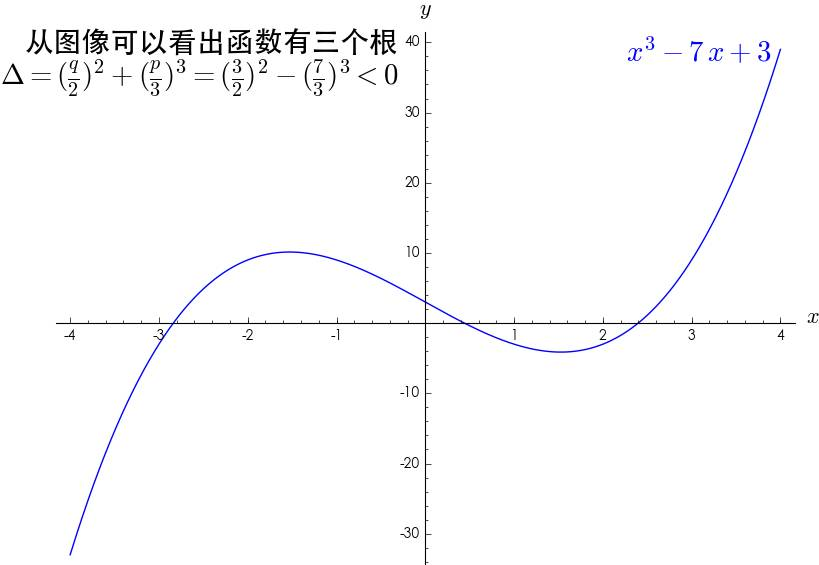### 2.4 虚数真实存在的理由

#### 2.4.1 $f(x)=sin(x)$泰勒展开

$f(x)=sin(x)$的收敛性在 $x=0$点泰勒展开: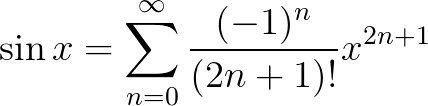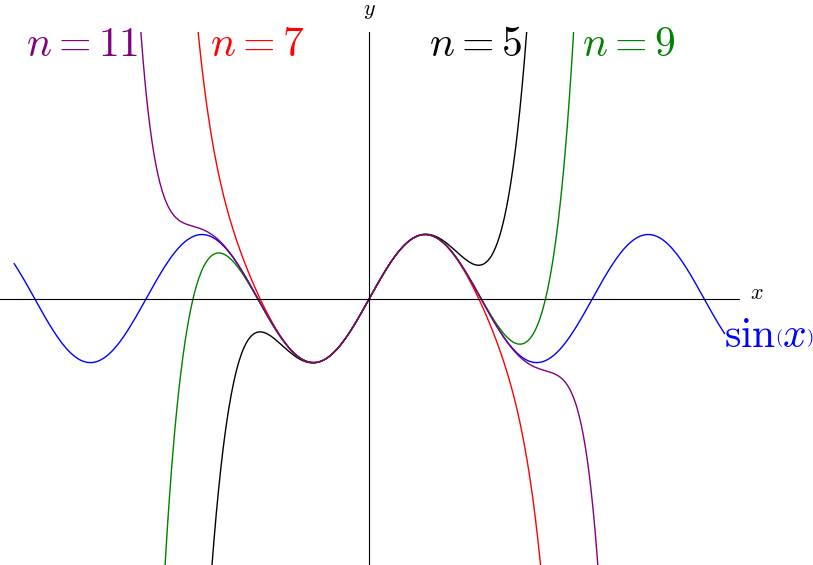$sin(x)$ 的泰勒级数在整个实数范围收敛，展开的阶数越多，对原函数的贴合就越好。

#### 2.4.2 $f(x)=\frac{1}{1-x}$的收敛性

$f(x)=\frac{1}{1-x}$的收敛性在 $x=0$ 点泰勒展开，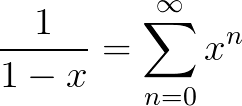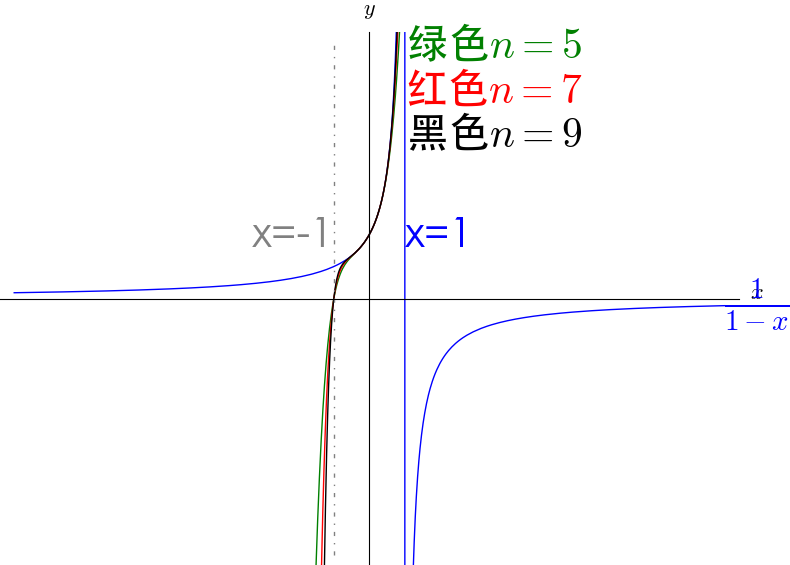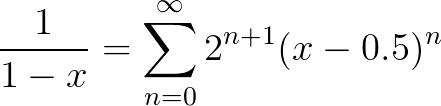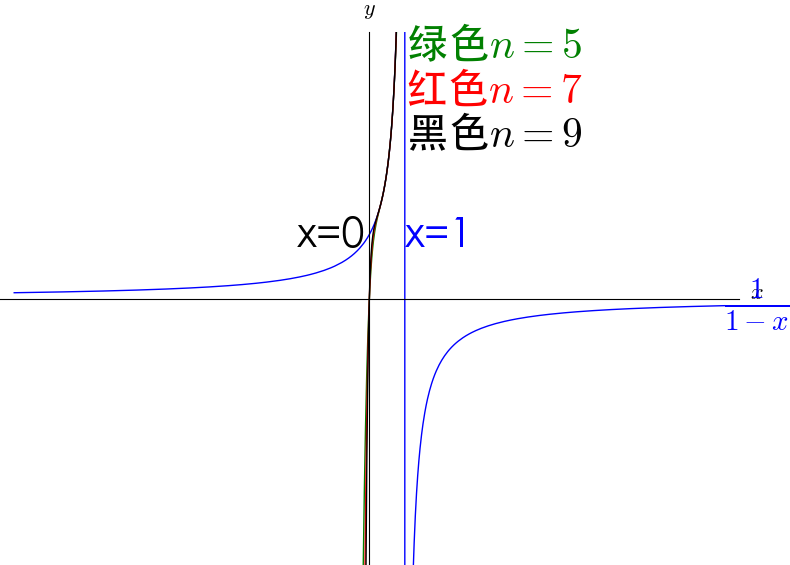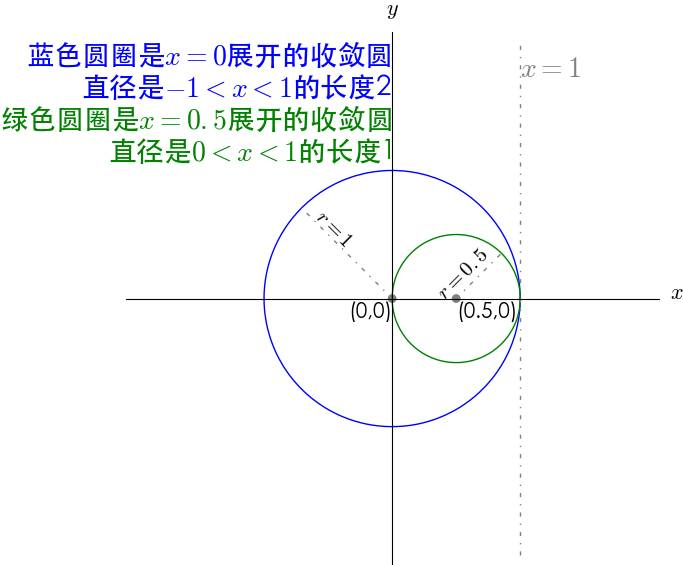#### 2.4.3 $f(x)=\frac{1}{1+x^2}$的收敛性

$f(x)=\frac{1}{1+x^2}$的收敛性在x=0 点泰勒展开，